# Heaviside step distribution

The Heaviside step distribution is defined by (Abramowitz and Stegun Eq. 29.1.3, p. 1020):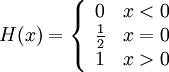$H(x) = \left\{ \begin{array}{ll} 0 & x < 0 \\ \frac{1}{2} & x=0\\ 1 & x > 0 \end{array} \right.$

Note that other definitions exist at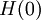$H(0)$, for example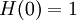$H(0)=1$. In the famous Mathematica computer package$H(0)$ is unevaluated.

## Differentiating the Heaviside distribution

At first glance things are hopeless: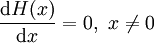$\frac{{\rm d}H(x)}{{\rm d}x}= 0, ~x \neq 0$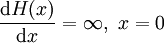$\frac{{\rm d}H(x)}{{\rm d}x}= \infty, ~x = 0$

however, lets define a less brutal jump in the form of a linear slope such that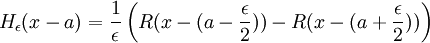$H_{\epsilon}(x-a)= \frac{1}{\epsilon}\left( R(x - (a-\frac{\epsilon}{2})) - R (x - (a+\frac{\epsilon}{2}))\right)$

in the limit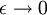$\epsilon \rightarrow 0$ this becomes the Heaviside function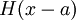$H(x-a)$. However, lets differentiate first: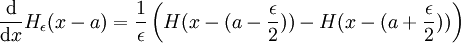$\frac{{\rm d}}{{\rm d}x} H_{\epsilon}(x-a)= \frac{1}{\epsilon}\left( H(x - (a-\frac{\epsilon}{2})) - H (x - (a+\frac{\epsilon}{2}))\right)$

in the limit this is the Dirac delta distribution. Thus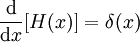$\frac{{\rm d}}{{\rm d}x} [H(x)]= \delta(x)$.